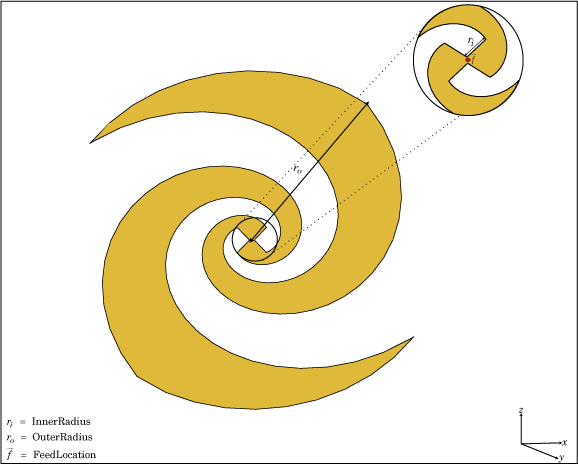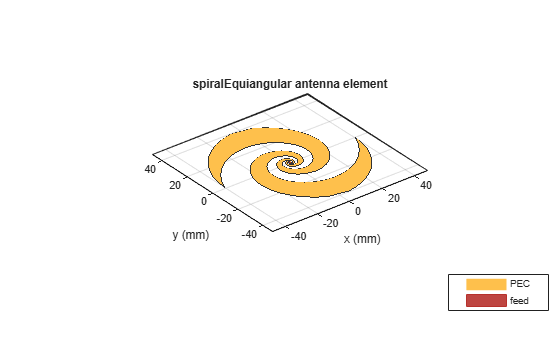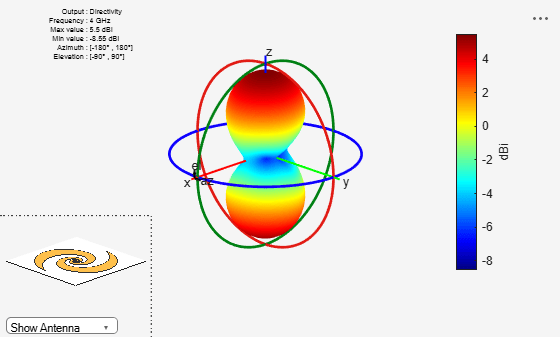# spiralEquiangular

Create equiangular spiral antenna

## Description

The `spiralEquiangular` object is a planar equiangular spiral antenna on the xy- plane. The equiangular spiral is always center fed and has two arms. The field characteristics of the antenna are frequency independent. A realizable spiral has finite limits on the feeding region and the outermost point of any arm of the spiral. This antenna exhibits a broadband behavior. The outer radius imposes the low frequency limit and the inner radius imposes the high frequency limit. The arm radius grows linearly as a function of the winding angle. As a result, outer arms of the spiral are shaped to minimize reflections.

The equation of the equiangular spiral is:

`$r={r}_{0}{e}^{a\varphi }$`

, where:

• r0 is the starting radius

• a is the growth rate

• ϕ is the winding angle of the spiral## Creation

### Syntax

``se = spiralEquiangular``
``se = spiralEquiangular(Name,Value)``

### Description

``` `se = spiralEquiangular` creates a planar equiangular spiral in the xy- plane. By default, the antenna operates over a broadband frequency 4–10 GHz.```

example

``` `se = spiralEquiangular(Name,Value)` creates an equiangular spiral antenna, with additional properties specified by one, or more name-value pair arguments. `Name` is the property name and `Value` is the corresponding value. You can specify several name-value pair arguments in any order as `Name1`, `Value1`,``` ...```, `NameN`, `ValueN`. Properties not specified retain their default values.```

## Properties

expand all

Equiangular spiral growth rate, specified as a scalar.

Example: `'GrowthRate',1.2`

Data Types: `double`

Inner radius of spiral, specified as a scalar in meters.

Example: `'InnerRadius',1e-3`

Data Types: `double`

Outer radius of spiral, specified as a scalar in meters.

Example: `'OuterRadius',1e-3`

Data Types: `double`

Direction of spiral turns (windings), specified as `'CW'` or `'CCW'`.

Example: `'WindingDirection'`,`'CW'`

Data Types: `char`

Type of the metal used as a conductor, specified as a metal material object. You can choose any metal from the `MetalCatalog` or specify a metal of your choice. For more information, see `metal`. For more information on metal conductor meshing, see Meshing.

Example: ```m = metal('Copper'); 'Conductor',m```

Example: ```m = metal('Copper'); ant.Conductor = m```

Lumped elements added to the antenna feed, specified as a lumped element object. For more information, see `lumpedElement`.

Example: `'Load',lumpedelement`. `lumpedelement` is the object for the load created using `lumpedElement`.

Example: ```se.Load = lumpedElement('Impedance',75)```

Tilt angle of the antenna, specified as a scalar or vector with each element unit in degrees. For more information, see Rotate Antennas and Arrays.

Example: `'Tilt',90`

Example: `ant.Tilt = 90`

Example: `'Tilt',[90 90]`,`'TiltAxis',[0 1 0;0 1 1]` tilts the antenna at 90 degrees about the two axes defined by the vectors.

Note

The `wireStack` antenna object only accepts the dot method to change its properties.

Data Types: `double`

Tilt axis of the antenna, specified as:

• Three-element vector of Cartesian coordinates in meters. In this case, each coordinate in the vector starts at the origin and lies along the specified points on the X-, Y-, and Z-axes.

• Two points in space, each specified as three-element vectors of Cartesian coordinates. In this case, the antenna rotates around the line joining the two points in space.

• A string input describing simple rotations around one of the principal axes, 'X', 'Y', or 'Z'.

Example: `'TiltAxis',[0 1 0]`

Example: `'TiltAxis',[0 0 0;0 1 0]`

Example: `ant.TiltAxis = 'Z'`

Note

The `wireStack` antenna object only accepts the dot method to change its properties.

Data Types: `double`

## Object Functions

 `show` Display antenna or array structure; display shape as filled patch `info` Display information about antenna or array `axialRatio` Axial ratio of antenna `beamwidth` Beamwidth of antenna `charge` Charge distribution on metal or dielectric antenna or array surface `current` Current distribution on metal or dielectric antenna or array surface `design` Design prototype antenna or arrays for resonance around specified frequency `efficiency` Radiation efficiency of antenna `EHfields` Electric and magnetic fields of antennas; Embedded electric and magnetic fields of antenna element in arrays `impedance` Input impedance of antenna; scan impedance of array `mesh` Mesh properties of metal or dielectric antenna or array structure `meshconfig` Change mesh mode of antenna structure `optimize` Optimize antenna or array using SADEA optimizer `pattern` Radiation pattern and phase of antenna or array; Embedded pattern of antenna element in array `patternAzimuth` Azimuth pattern of antenna or array `patternElevation` Elevation pattern of antenna or array `rcs` Calculate and plot radar cross section (RCS) of platform, antenna, or array `returnLoss` Return loss of antenna; scan return loss of array `sparameters` Calculate S-parameter for antenna and antenna array objects `vswr` Voltage standing wave ratio of antenna

## Examples

collapse all

Create and view an equiangular spiral antenna with 0.35 growth rate, 0.65 mm inner radius and 40 mm outer radius.

```se = spiralEquiangular('GrowthRate',0.35, 'InnerRadius',0.65e-3, ... 'OuterRadius',40e-3); show(se)```Plot the radiation pattern of equiangular spiral at a frequency of 4 GHz.

```se = spiralEquiangular('GrowthRate',0.35, 'InnerRadius',0.65e-3, ... 'OuterRadius',40e-3); pattern(se,4e9);```Dyson, J. The equiangular spiral antenna.” IRE Transactions on Antennas and Propagation. Vol.7, Number 2, pp. 181, 187, April 1959.

 Nakano, H., K.Kikkawa, N.Kondo, Y.Iitsuka, J.Yamauchi. “Low-Profile Equiangular Spiral Antenna Backed by an EBG Reflector.” IRE Transactions on Antennas and Propagation. Vol. 57, No. 25, May 2009, pp. 1309–1318.

 McFadden, M., and Scott, W.R. “Analysis of the Equiangular Spiral Antenna on a Dielectric Substrate.” IEEE Transactions on Antennas and Propagation. Vol. 55, No. 11, Nov. 2007, pp. 3163–3171.

 Violakis, John Antenna Engineering Handbook, 4th Ed., McGraw-Hill.